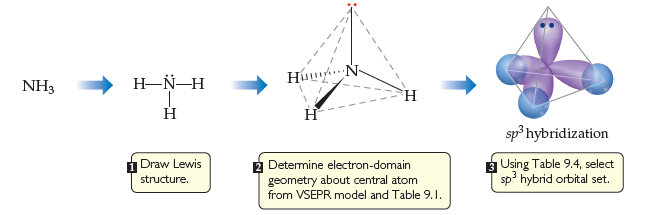# Problem: How would we modify the figure if we were looking at PH3 rather than NH3?

###### FREE Expert Solution

We are asked how would we modify the figure if we were looking at PH3 rather than NH3.

Compare the molecules:

• We can observe that we both have 3 H on each molecule.
• If we look at the periodic table, we can see that P and N are both on group 5A.###### Problem Details

How would we modify the figure if we were looking at PH3 rather than NH3?